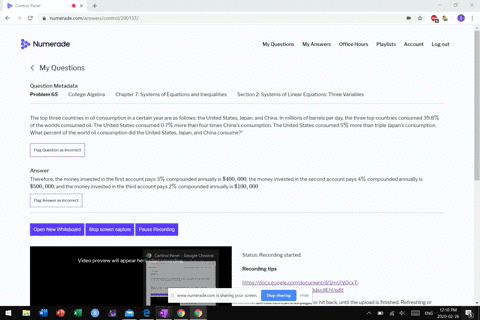Sign up for our free STEM online summer camps starting June 1st!View Summer Courses### The top three countries in oil consumption in a c…

01:22
MB

Need more help? Fill out this quick form to get professional live tutoring.

Get live tutoring
Problem 64

You inherit one hundred thousand dollars. You invest it all in three accounts for one year. The first account pays 4$\%$ compounded annually, the second account pays 3$\%$ compounded annually, and the third account pays 2$\%$ compounded annually. After one year, you earn $\$ 3,650$in interest. If you invest five times the money in the account that pays 4$\%$compared to$3 \%,$how much did you invest in each account? Answer Therefore, the money invested in first account that paid 4$\%$simple interest is$\$25,500$, the money invested in second account that paid 3$\frac{1}{8} \%$ simple interest is $\$ 44,000$and the money invested in third account that paid 2$\frac{1}{2} \%$simple interest is$\$11,000$.

## Discussion

You must be signed in to discuss.

## Video Transcript

all right. So, uh, lucky you you inherited $100,000 You smartly decided to invest it into three accounts. One face or percent. One phase, 3% 1 It's 2%. No way would put it on 4% account, but, um, after one year, you have$3650 and we're also told that mountain 4% account is five times the amount that's in 3%. Really? Stay put most of their money in the world right. So $363,650 into step one year. So you inherited$100,000. So if we add up the investments here, that should be 100,000. So you start off by saying that X plus y Z is equal to 100,000. And then we know the interest after one year is 33,650. So that would be, um, we know or ex plus 0.3. Why? Plus point to Z that equals three thousands. The final one is the money that's in before percent account equals five times the amount of money to the 3% the count. All right. Okay, So first things first, Let's go ahead and substitute are five wife for our exes in these equations. So my first equation becomes five. Why? Why? Why us? Why? So maybe six? Why plus z equals 100,000 and then five times 50.4 is, uh or is put to So I've got, um uh, 0.2. Why here in 103 So 123 Why? First point? Oh, to your ex in C 2 3000 All right. And I want to try the cancel. These things are canceling variable. All right, so, um, stake here. This is all right. Um Okay, so let's vote by this equation right here. Bye. Fire that make it from one. So 50. Okay, so 0.23 times 50 people's, 11.5. Why? And then one. No, two times 50. I'm supposed to. People wonder it does equals then 3600. All right, ISS, turn 82,500. All right. And we can subtract these two equations right here. It's attractive. Let's do bottom minus. Stop. Attractive in that way. So 11.5 minus six is five fires, so I get five bye. Why equals now? The disease. Cancel. So then I have to do 182,500. Minus 100,000. You got 82,000. Didn't divide that by 5.5. They get \$15,000 in accounts. Why? Okay, it's That means X is gonna be five times that of him. 75,000. Okay. And so so far, we've used up 90,000. That means total investment was hundreds. See? Asked of you.